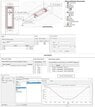# Optimetrics simulation of a coil and moving permanent magnet in Ansys Maxwell

Y_G
I'm trying to simulate a Magnetostatic problem. In my model there are two coils and a reciprocating permanent magnet. I defined a parameter, Xpm (movement of permanent magnet) in x direction to imply the reciprocating movement of permanent magnet like a piston. Xpm = f(t). I defined "t" as sweep parameter. When the time changes and the magnet moves back and forth, the amount of Flux will change in the iron core of the coil. Obviously, because of magnetostatic simulation, I can't obtain induced current in coils.
Is it possible to obtain how much voltage induced in coils by moving the magnet?

The picture I attached shows how I setup the model. After calculation of "MagFlux" in Optimetrics section, I used excel to calculate how much voltage induced to the coil by using this equation: V = -d(phi)/dt.

Is that correct what I've done?

#### Attachments

•Sliding-Generator.jpg
43.6 KB · Views: 75

Is it possible to obtain how much voltage induced in coils by moving the magnet?
Welcome to PF.
Yes. How many turns of wire are wound on each coil ?

Y_G
Thank You, 2000 turns for each coil. I did this modeling in two ways. 2000 and 1000 turns. In the first case, the amount of magnetic flux obtained was higher. To calculate the induced current in the coil, assuming the AWG24 wire, I calculated the length of the coil and obtained its resistance. Finally, the induced current was obtained with the relation V = RI.

Should the coil be modeled as a closed circuit, with current and partial magnetic cancellation, or should you model it open circuit for voltage?
That will depend on whether you are generating power or measuring magnet position and velocity. Why are you doing this design?

Y_G
This is a kind of generator, If we could say. Sliding Generator. So I think, It should be modeled open circuit for voltage. Actually I defined another parameter for calculating force on moving magnet in x-direction. But I'm aware because of magnetostatic simulation the force may different from real case scenario.
I hope I understand what you mean.

Staff Emeritus
This is a kind of generator, If we could say. Sliding Generator. So I think, It should be modeled open circuit for voltage.
That's contradictory. A generator does not generate open circuit.

Y_G
That's contradictory. A generator does not generate open circuit.
Thanks for replying. I only defined stranded coils with terminals. I don't know if it could be a open circuit or close circuit.

Staff Emeritus
So are you just experimenting to see what you can do?

If so, I suggest starting open circuit, and measuring what voltage you get. Maybe you can see how the voltage varies as the magnet makes a complete revolution.

•Y_G
This is fundamentally an inefficient generator topology because the magnetic field through the coils does not change polarity.

The PM flux is divided into two paths. The difference between maximum and minimum flux comes from the series length of the sides that are part of the two magnetic circuits through the coils. Is it possible to increase the flux change, and so the output, by reducing the mass of magnetic material in the side rails.

The cost will be high because;
1. The PM must slide between the rails with a minimum gap.
2. There are two coils.

•Y_G
Y_G
So are you just experimenting to see what you can do?

If so, I suggest starting open circuit, and measuring what voltage you get. Maybe you can see how the voltage varies as the magnet makes a complete revolution.
Thanks

Y_G
This is fundamentally an inefficient generator topology because the magnetic field through the coils does not change polarity.

The PM flux is divided into two paths. The difference between maximum and minimum flux comes from the series length of the sides that are part of the two magnetic circuits through the coils. Is it possible to increase the flux change, and so the output, by reducing the mass of magnetic material in the side rails.

The cost will be high because;
1. The PM must slide between the rails with a minimum gap.
2. There are two coils.
Thank you so much for your help. Despite of ordinary generator, maybe it could be possible to omit the gap between rails and PM. Also I'm curious about reducing the force on PM. If the force is reduced to a minimum level the efficiency of the generator will increase significantly.

•